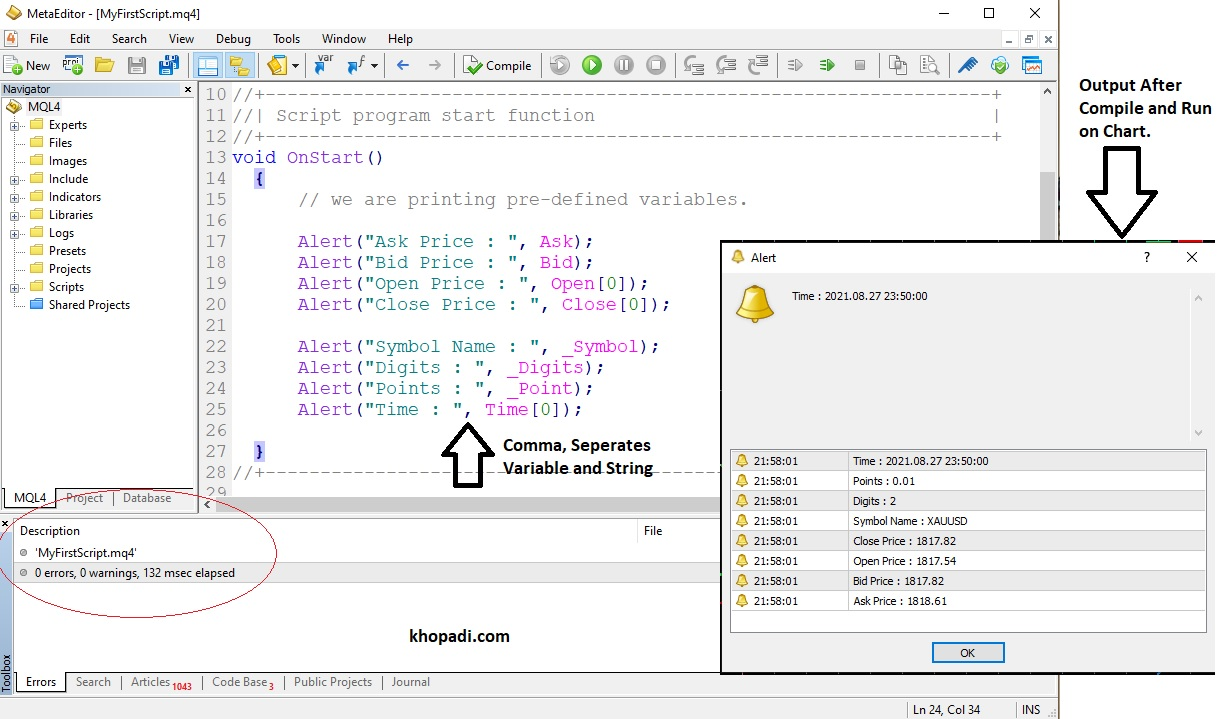# ⚡ Variables in MQL

## Variables in MQL ⚡

Each Computer Programming language has the option to store dynamic data. which can be used for further operations.

Data:- Meaningful information Set, for desired System Implementation.
For Example, If you are creating a Trading System then Open Price, Close Price, Number of Bars in Chart, Current value of Spread, etc are data. which you can use for the Implementation of the Trading Strategy.

## What is Variable in MQL?

Variable is the storage container that is used to save the predefined or user data. The value of a variable can be changed as per requirement.

In MetaQuotes Language (MQL), There are Two Categories of Variables.
1. Pre-Defined Variables by MQL
2. User-Defined Variables

### Pre-Defined Variable

The Variable, which is already defined by the creators of MQL. So that you can directly use the Power of MQL and can develop your trading system in a short period of time. In the following, I have listed only important pre-defined variables. which I use in future tutorials.
you can find the complete list here.

No Variable Name Use of Pre Defined Variable
2. Bid Current Selling Price (Buyer's Price)
3. Bars Number of Total Bars Available on Current Chart
4. High Collection Array of High Prices (Bar Number wise)
5. Low Collection Array of Low Prices (Bar Number wise)
6. Open Collection Array of Open Prices (Bar Number wise)
7. Close Collection Array of Close Prices (Bar Number wise)
8. Time Collection Array of Open Time of Each Bar
9. Volume Collection Array of Tick Volume of Each Bar
10. _Point Point Size available in Selected Currency Chart
11. _Digits Number of digits after Decimal Point in Selected Currency
12. _Symbol Currency Symbol Name which is selected on Chart
13. _Period Timeframe of Selected Chart
14. _LastError Code of Last known Error generated

I have selected the "XAUUSD" chart on the 5-Minute Timeframe. so that you can see the output of the following code.Pre-Defined Variable in MQL (Click on Image for Zoom In)

Source Code
`void OnStart()  {      // we are printing pre-defined variables.                  Alert("Ask Price : ", Ask);             Alert("Bid Price : ", Bid);      Alert("Open Price : ", Open);      Alert("Close Price : ", Close);            Alert("Symbol Name : ", _Symbol);             Alert("Digits : ", _Digits);      Alert("Points : ", _Point);      Alert("Time : ", Time);        }`

### User-Defined Variable

The Variable, which is used to store the data defined by the user.

For Example:-
You want to add Two Numbers and display the sum of these two numbers after storing the value of the sum.

Then, Mathematical Logic says,

You need x,y Two variables, and sum as the result variable (if you are familiar with basic algebra maths)

Suppose:- x = 10  and  y = 20
Thus :-   sum = x + y
and you can simply pass the variable "sum" in Print Function or Alert Function as we have used in our previous MQL Tutorials of ⚡ Basic "Hello World Program" Execution in MQL4

For Programming Implementation, you do something deeper.

#### How to declare a user-defined variable in MQL?

For Declaration of Variable in MQL Programming, we use the following syntax:-

Syntax for declaration of Variable Name

DataType VariableName = InitialValue  ; (Semi-Colon)

Example,

DataType = int (Integer Number)
Variable Name = x
Initial Value = 10

Then Final Syntax will be

int x = 10 ;

Note:- Semi-Colon is necessary to put at the End of each Statement of the MQL program. It is called Statement Terminator. It is just like a full stop which you put at the end of the English Language Sentence.

Source Code
`void OnStart()  {      // we are printing user-defined variables.                  int x = 10 ;      int y = 20 ;            int sum = x + y ;            Alert("Sum : ", sum) ;  }`

## Rules for Variable Name in MQL

You must take precautions for the declaration of a Variable Name. There are some rules related to Variable Names. The variable name is also called an Identifier Name.
1. Variable Name can be a combination of Letters, Digits, and Underscore ( _ ).
3. Variable names are case-sensitive, which means x and X both are different as per variable rules.
4. A variable name can not contain any Space.
5. Variable Name must not be a pre-defined Identifier (Which is already defined by Language creators. For Example, if, else, switch, print, Ask, _Digit, etc.)

• numX
• Num1
• _Num
• _numX
• Num1_numX

• 1num
• 23num
• 7x_
• Raj Kumr